# Hopf-Tsuji-Sullivan theorem

This theorem establishes the equivalence of several characterizations of "smallness" of a Riemannian manifold of constant negative curvature, or, more generally, of a discrete groupof isometries of the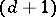-dimensional hyperbolic space(cf. also Discrete group of transformations).

Denote by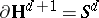the sphere at infinity (the visibility sphere), of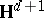, and fix an origin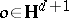. A point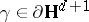is called a radial limit point of the groupif there exists a number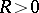such that the-neighbourhood of the geodesic ray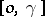contains infinitely many points from the orbit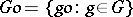. The set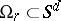of all radial limit points is called the radial limit set of. Alternatively, let the shadowof the ball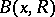of radiuscentred at a point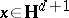be the set of end-points of all geodesic rays which are issued fromand intersect. Thenif and only if there is ansuch thatbelongs to an infinite number of shadows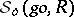,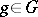.

The following conditions are equivalent:

1) The Poincaré series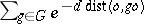diverges, where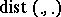is the Riemannian distance on.

2) The quotient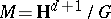has no Green function, i.e.,has no non-constant negative subharmonic functions (cf. also Subharmonic function), or, equivalently, the Brownian motion onis recurrent.

3) The complement of the radial limit set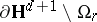has Lebesgue measure zero.

4) The geodesic flow onis ergodic with respect to the Liouville-invariant measure (the one determined by the Riemannian volume).

5) The action ofon the product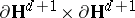is ergodic with respect to the Lebesgue measure.

Usually the term "Hopf–Tsuji–Sullivan theorem" is applied to the equivalence of 1), 3) and 4). For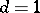the implication 3)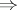4) was first proved by E. Hopf [a1], [a2], and the implications 4)1)3) by M. Tsuji, see [a3]. Tsuji's proof is essentially-dimensional, as it uses complex function theory, whereas Hopf's argument easily carries over to the higher-dimensional case. D. Sullivan [a4] used an entirely different way for proving the chain of implications 4)3)1)4) for an arbitrary dimension.

The equivalence of 1) and 2) follows from the asymptotic equivalence of the Green function onto, whereas the equivalence of 3), 4) and 5) is a much more general fact, see Hopf alternative. Sullivan's idea was to deduce the implication 2)5) from general properties of recurrent Markov operators. On the other hand, the implication 3)1) is an easy corollary of the estimate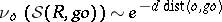, where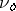is the image of the Lebesgue measure on the unit tangent sphere at the point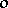under the exponential mapping (a particular case of the Sullivan shadow lemma).

Ergodicity of the action ofon(i.e., absence of bounded harmonic functions on) is weaker than 5). For Riemannian surfaces the implication "no Green function""no non-constant bounded harmonic functions" is known as the Myrberg theorem, see [a5]. In probabilistic terms, this implication can be reformulated as "ergodicity of the time shift in the bilateral path space""ergodicity of the time shift in the unilateral path space" , or just that recurrence of the Brownian motionimplies absence of non-constant bounded harmonic functions [a6]. The latter reformulation allows one to construct examples of discrete groups of isometries ofwhose action on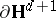is ergodic and onis not, in a much simpler way than original Riemann surface examples, [a7].

An analogue of the Hopf–Tsuji–Sullivan theorem for the invariant measure of the geodesic flow corresponding to the Patterson–Sullivan measure onwas proved in [a8], see also [a9]. In this setup, condition 1) is replaced by divergence of the Poincaré series at the critical exponent of the group.

In the non-constant curvature case, generalizations of the Hopf–Tsuji–Sullivan theorem were obtained in [a6] for the harmonic invariant measure of the geodesic flow and in [a10] for the Patterson–Sullivan measure.

How to Cite This Entry:
Hopf-Tsuji-Sullivan theorem. Encyclopedia of Mathematics. URL: http://encyclopediaofmath.org/index.php?title=Hopf-Tsuji-Sullivan_theorem&oldid=22593
This article was adapted from an original article by V.A. Kaimanovich (originator), which appeared in Encyclopedia of Mathematics - ISBN 1402006098. See original article